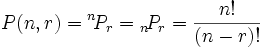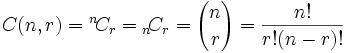# Permutation & Combination

Factorial Notation
Let n be a positive integer. Factorial of n denoted by n! and is defined as
n! = n(n - 1)(n - 2)(n-3) ... 3.2.1.
Examples:
We define 0! = 1.
2! = 2.1 = 2
3! = 3.2.1 = 6
4! = 4.3.2.1 = 24
5! = 5.4.3.2.1 = 120
So on......

Permutations (Arrangements
The different arrangements of a given number or things by taking some or all at a time, are called permutation.

Examples:
1. All permutations (or arrangements) made with the letters a, b, c by taking two at a time are (ab, ba, ac, ca, bc, cb).
2. All permutations made with the letters a, b, c taking all at a time are:
( abc, acb, bac, bca, cab, cba).

Number of Permutations:
Number of all permutations of n things, taken r at a time, is given by:nPr = n(n - 1)(n - 2) ... (n - r + 1)

Examples:
• 6P2 = (6 x 5) = 30.
• 7P3 = (7 x 6 x 5) = 210.
Cor. number of all permutations of n things, taken all at a time = n!.

Note: If there are n subjects of which p1 are alike of one kind; p2 are alike of another kind;p3 are alike of third kind and so on and pr are alike of rth kind,
such that (p1 + p2 + ... pr) = n.
 Then, number of permutations of these n objects is = n! (p1!).(p2)!.....(pr!)

Combinations
Each of the different groups or selections which can be formed by taking some or all of a number of objects is called a combination.

Examples:
• Suppose we want to select two out of three boys A, B, C. Then, possible selections are AB, BC and CA.
Note: AB and BA represent the same selection.
• All the combinations formed by a, b, c taking ab, bc, ca.
• The only combination that can be formed of three letters a, b, c taken all at a time is abc.
• Various groups of 2 out of four persons A, B, C, D are: AB, AC, AD, BC, BD, CD.
Note: that ab ba are two different permutations but they represent the same combination.

Number of Combinations:
The number of all combinations of n things, taken r at a time is:Note:
1.           nCn = 1 and nC0 = 1.
2.         nCr = nC(n - r)

Examples:

 11C4 = (11 x 10 x 9 x 8) = 330. (4 x 3 x 2 x 1)
 16C13 = 16C(16 - 13) = 16C3 = 16 x 15 x 14 = 16 x 15 x 14 = 560. 3! 3 x 2 x 1
Choosing 3 balls out of 16, or choosing 13 balls out of 16 have the same number of combinations.
 16! = 16! = 16! = 560 3!(16-3)! 13!(16-13)! 3!×13!Thanks for reading Permutation & Combination

←Previous
« Prev Post
Next→
Next Post »

Video Lectures